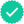#### Related questions

Question

Use Lagrange multipliers to find the given extremum. Assume that x and y are positive.

Minimize f(x, y) = x2 − 4x + y2 − 16y + 42

Constraint: x + y = 24

Minimum of f(x, y) =

at (x, y) =
Expert SolutionKnowledge BoosterRecommended textbooks for you
•Algebra & Trigonometry with Analytic Geometry
Algebra
ISBN:9781133382119
Author:Swokowski
Publisher:Cengage
•Algebra & Trigonometry with Analytic Geometry
Algebra
ISBN:9781133382119
Author:Swokowski
Publisher:Cengage